### Home > CALC > Chapter 4 > Lesson 4.1.2 > Problem4-18

4-18.
1. Evaluate the following definite integrals. Homework Help ✎

1.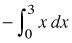2.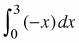3.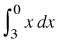4.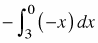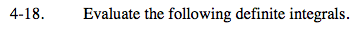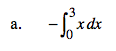Ignoring the negative for the moment, sketch and shade

$\int_{0}^{3}xdx.$

The area under the curve is a triangle with base 3 and height 3. Find the area ... then make it negative.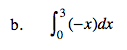The area of the triangle is below the x-axis. Does this make it positive or negative?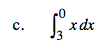Notice that the integrand is the same as in part (a); but the bounds have flipped, causing you to look at the base of the triangle from right to left. This will make the area negative.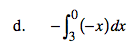Think through all the negatives and reverse bounds. Is there a way to rewrite this integral so it will be simpler to sketch and evaluate?